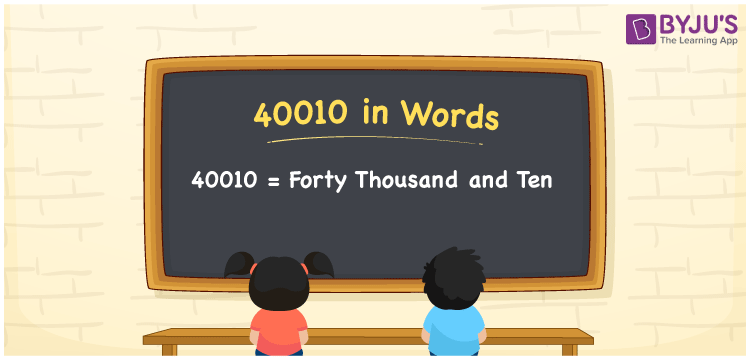# 40010 in Words

In English words, the number name of 40010 is “forty thousand and ten”. The number 40010 is a five-digit number and its place values are useful in writing the number names. 40010 lies after the natural number 40009 but before 40011. Also, 40010 is a cardinal number.

 40010 in Words: Forty Thousand and Ten. Forty Thousand and Ten in Numerical Form: 40010.

## 40010 in English Words## How to Write 40010 in Words?

The place value table for the numeral 40010 up to five digits is given below:

 Ten-thousands Thousands Hundreds Tens Ones 4 0 0 1 0

The expanded form of 40010 is as follows:

= 4 × Ten thousand + 0 × Thousand + 0 × Hundred + 1 × Ten + 0 × One

= 4 × 10000 + 0 × 1000 + 0 × 100 + 1 × 10 + 0 × 1

= 40000 + 10

= 40010

= Forty thousand and ten

Hence, 40010 in words is forty thousand and ten.

40010 in words – Forty thousand and ten

Is 40010 an odd number? – No

Is 40010 an even number? – Yes

Is 40010 a perfect square number? – No

Is 40010 a perfect cube number? – No

Is 40010 a prime number? – No

Is 40010 a composite number? – Yes

## Frequently Asked Questions on 40010 in Words

Q1

### Write 40010 in words.

40010 in words is forty thousand and ten.

Q2

### Simplify 40000 + 10, and express it in words.

Simplifying 40000 + 10, we get 40010. Hence, 40010 in words is forty thousand and ten.

Q3

### Is 40010 an even number?

Yes, 40010 is an even number.